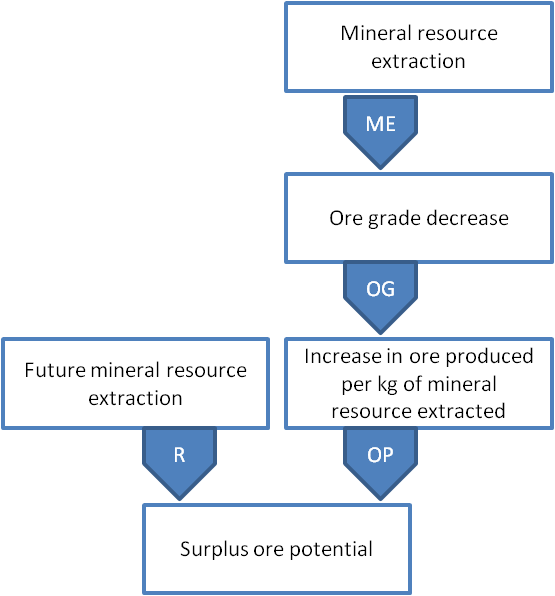a spatially differentiated life cycle impact assessment method# Mineral Resource Scarcity

#### Resources

###### Mineral Resource Scarcity

Metals and mineral resources are key raw materials in many industrial sectors, such as construction, energy, and transportation. Although recovery of many metals in being done through recycling, this still doesn’t meet the demand so extraction of primary resources is needed.

#### Cause-effect pathway

The impact model is addressing the future amount of average extra ore to be mined and consequent effects on the Area of protection ‘Resources’ caused by mineral resource consumption.#### Modeling approach

An average approach is used to calculate the characterization factors. By calculating on basis of cumulative grade-tonnage relationships the increase in ore amount for all future mineral extraction and then dividing it by the future mineral extraction, average CFs are derived. These CFs are used to assess the potential impacts of mineral resource extraction worldwide.

#### Value choices

• Time horizon: infinite
• Maximum amount of a mineral resource to be extracted: two different reserve estimates were applied in the calculations, reserves and ultimate recoverable resource
• The choice of discounting: no discounting to future effects is applied

Not applicable

#### Characterisation factors

The equation for calculating the characterisation factor (CF), expressed as the Surplus Ore Potential (SOP), is defined as the extra amount of ore produced in the future per unit of mineral extracted (kgore/kgx) is:

$$\mathsf{CF_{x}=\frac{\int_{CME_x}^{MME_x}\left(\Delta OP_x\right)\mathit{d}CME_x}{MME_x-CME_x}}$$

where OPx is the ore produced per amount of mineral resource x extracted (kgore/kgx), MMEx (kgore/kgx) is the maximum amount to be extracted of that mineral, and CMEx is the cumulative amount of mineral x currently extracted.